# Square root

Square root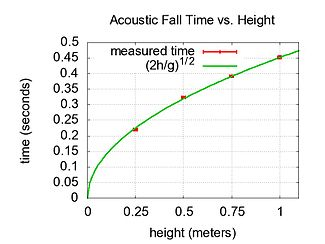Measured fall time of a small steel sphere falling from various heights. The data is in good agreement with the predicted fall time of$\sqrt{2h/g}$, where h is the height and g is the acceleration of gravity.

In mathematics, a square root of a number x is a number r such that r2 = x, or, in other words, a number r whose square (the result of multiplying the number by itself, or r × r) is x. For example, 4 is a square root of 16 because 42 = 16.

Every non-negative real number x has a unique non-negative square root, called the principal square root, denoted by a radical sign as$\sqrt{x}$. For positive x, the principal square root can also be written in exponent notation, as x1/2. For example, the principal square root of 9 is 3, denoted$\sqrt{9} \ = \ 3$, because 32 = 3 × 3 = 9 and 3 is non-negative. Although the principal square root of a positive number is only one of its two square roots, the designation "the square root" is often used to refer to the principal square root.

Every positive number x has two square roots:$\sqrt{x}$, which is positive, and$-\sqrt{x}$, which is negative. Together, these two roots are denoted$\pm\sqrt{x}$ (see ± shorthand). Square roots of negative numbers can be discussed within the framework of complex numbers. More generally, square roots can be considered in any context in which a notion of "squaring" of some mathematical objects is defined (including algebras of matrices, endomorphism rings, etc.)

Square roots of integers that are not perfect squares are always irrational numbers: numbers not expressible as a ratio of two integers (that is to say they cannot be written exactly as m/n, where m and n are integers). This is the theorem Euclid X, 9 almost certainly due to Theaetetus dating back to circa 380 BC. The particular case$\sqrt{2}$ is assumed to date back earlier to the Pythagoreans and is traditionally attributed to Hippasus. It is exactly the length of the diagonal of a square with side length 1.

The term whose root is being considered is known as the radicand. The radicand is the number or expression underneath the radical sign. For example, in the expression$\sqrt{ab+2}$, ab + 2 is the radicand.

## Properties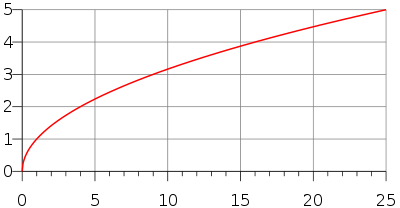The graph of the function$f(x) = \sqrt{x}$, made up of half a parabola with a vertical directrix.

The principal square root function$f(x) = \sqrt{x}$ (usually just referred to as the "square root function") is a function that maps the set of non-negative real numbers onto itself. In geometrical terms, the square root function maps the area of a square to its side length.

The square root of x is rational if and only if x is a rational number that can be represented as a ratio of two perfect squares. (See square root of 2 for proofs that this is an irrational number, and quadratic irrational for a proof for all non-square natural numbers.) The square root function maps rational numbers into algebraic numbers (a superset of the rational numbers).

For all real numbers x$\sqrt{x^2} = \left|x\right| = \begin{cases} x, & \mbox{if }x \ge 0 \\ -x, & \mbox{if }x < 0. \end{cases}$     (see absolute value)

For all non-negative real numbers x and y,$\sqrt{xy} = \sqrt x \sqrt y$

and$\sqrt x = x^{1/2}.$

The square root function is continuous for all non-negative x and differentiable for all positive x. If f denotes the square-root function, its derivative is given by:$f'(x) = \frac{1}{2\sqrt x}.$

The Taylor series of √1 + x about x = 0 converges for |x| ≤ 1 and is given by$\sqrt{1 + x} = \sum_{n=0}^\infty \frac{(-1)^n(2n)!}{(1-2n)(n!)^2(4^n)}x^n = 1 + \textstyle \frac{1}{2}x - \frac{1}{8}x^2 + \frac{1}{16} x^3 - \frac{5}{128} x^4 + \dots,\!$

which is a special case of a binomial series.

## Computation

Most pocket calculators have a square root key. Computer spreadsheets and other software are also frequently used to calculate square roots. Pocket calculators typically implement efficient routines to compute the exponential function and the natural logarithm or common logarithm, and use them to compute the square root of a positive real number x using the identity$\sqrt{x} = e^{(\ln x)/2}$ or$\sqrt{x} = 10^{(\log x)/2}.$

The same identity is exploited when computing square roots with logarithm tables or slide rules.

The most common iterative method of square root calculation by hand is known as the "Babylonian method" or "Heron's method" after the first-century Greek philosopher Heron of Alexandria, who first described it. The method uses the same iterative scheme as the Newton-Raphson process yields when applied to the function$y=f(x)=x^2-a$, using the fact that its slope at any point is$dy/dx=f'(x)=2x$, but predates it by many centuries. It involves a simple algorithm, which results in a number closer to the actual square root each time it is repeated. The basic idea is that if x is an overestimate to the square root of a non-negative real number a then$a/x\,$ will be an underestimate and so the average of these two numbers may reasonably be expected to provide a better approximation (though the formal proof of that assertion depends on the inequality of arithmetic and geometric means that shows this average is always an overestimate of the square root, as noted below, thus assuring convergence). To find x :

1. Start with an arbitrary positive start value x (the closer to the square root of a, the fewer iterations will be needed to achieve the desired precision).
2. Replace x by the average between x and a/x, that is:$(x + a/x) / 2\,$, representing the Newton-Raphson scheme$x_{n+1} = x_n - f(x_n)/f'(x_n)$ resulting in$x_{n+1} = (x_n+a/x_n)/2$,

(It is sufficient to take an approximate value of the average to ensure convergence)

1. Repeat step 2 until x and a/x are as close as desired.

If a is positive, the convergence is "quadratic," which means that in approaching the limit, the number of correct digits roughly doubles in each next iteration. If a = 0, the convergence is only linear.

Using the identity$\sqrt{a} = 2^{-n}\sqrt{4^n a},$

the computation of the square root of a positive number can be reduced to that of a number in the range [1, 4). This simplifies finding a start value for the iterative method that is close to the square root, for which a polynomial or piecewise-linear approximation can be used.

The time complexity for computing a square root with n digits of precision is equivalent to that of multiplying two n-digit numbers.

## Square roots of negative and complex numbers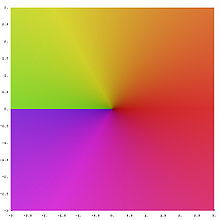Complex square root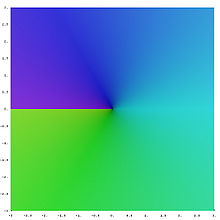Second leaf of the complex square root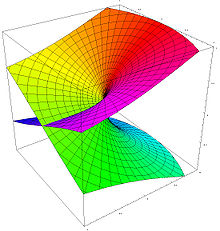Using the Riemann surface of the square root, one can see how the two leaves fit together

The square of any positive or negative number is positive, and the square of 0 is 0. Therefore, no negative number can have a real square root. However, it is possible to work with a more inclusive set of numbers, called the complex numbers, that does contain solutions to the square root of a negative number. This is done by introducing a new number, denoted by i (sometimes j, especially in the context of electricity where "i" traditionally represents electric current) and called the imaginary unit, which is defined such that i2 = –1. Using this notation, we can think of i as the square root of –1, but notice that we also have (–i)2 = i2 = –1 and so –i is also a square root of –1. By convention, the principal square root of –1 is i, or more generally, if x is any positive number, then the principal square root of –x is$\sqrt{-x} = i \sqrt x.$

The right side (as well as its negative) is indeed a square root of –x, since$(i\sqrt x)^2 = i^2(\sqrt x)^2 = (-1)x = -x.$

For every non-zero complex number z there exist precisely two numbers w such that w2 = z: the principal square root of z (defined below), and its negative.

### Square root of an imaginary number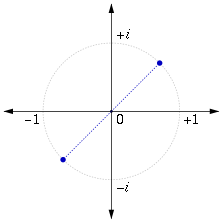The square roots of i in the complex plane

The square root of i is given by$\sqrt{i} = \frac{1}{2}\sqrt{2} + i\frac{1}{2}\sqrt{2} = \frac{\sqrt{2}}{2}(1+i).$

This result can be obtained algebraically by finding a and b such that$i = (a+bi)^2\,\!$

or equivalently$i = a^2 + 2abi - b^2.\,\!$

This gives the two simultaneous equations$\begin{cases} 2ab = 1\,\! \\ a^2 - b^2 = 0\,\! \end{cases}$

with solutions$a = b = \pm \frac{1}{\sqrt{2}}.$

The choice of the principal root then gives$a = b = \frac{1}{\sqrt{2}}.$

The result can also be obtained by using de Moivre's formula and setting$i = \cos\left (\frac{\pi}{2}\right ) + i\sin\left (\frac{\pi}{2}\right )$

which produces\begin{align} \sqrt{i} & = \left ( \cos\left ( \frac{\pi}{2} \right ) + i\sin \left (\frac{\pi}{2} \right ) \right )^{\frac{1}{2}} \\ & = \cos\left (\frac{\pi}{4} \right ) + i\sin\left ( \frac{\pi}{4} \right ) \\ & = \frac{1}{\sqrt{2}} + i\left ( \frac{1}{\sqrt{2}} \right ) = \frac{1}{\sqrt{2}}(1+i) . \\ \end{align}

### Principal square root of a complex number

To find a definition for the square root that allows us to consistently choose a single value, called the principal value, we start by observing that any complex number x + iy can be viewed as a point in the plane, (x, y), expressed using Cartesian coordinates. The same point may be reinterpreted using polar coordinates as the pair (r, φ), where r ≥ 0 is the distance of the point from the origin, and φ is the angle that the line from the origin to the point makes with the positive real (x) axis. In complex analysis, this value is conventionally written re. If$z=r e^{\varphi i} \text{ with } -\pi < \varphi \le \pi, \,$

then we define the principal square root of z as follows:$\sqrt{z} = \sqrt{r} \, e^{i \varphi / 2}.$

The principal square root function is thus defined using the nonpositive real axis as a branch cut. The principal square root function is holomorphic everywhere except on the set of non-positive real numbers (on strictly negative reals it isn't even continuous). The above Taylor series for √1 + x remains valid for complex numbers x with |x| < 1.

The above can also be expressed in terms of trigonometric functions:$\sqrt{r \left(\cos \varphi + i \, \sin \varphi \right)]} = \sqrt{r}[\cos \frac{\varphi}{2} + i \sin \frac{\varphi}{2}]. \,$

### Algebraic formula

When the number is expressed using Cartesian coordinates the following formula can be used for the principal square root:$\sqrt{x+iy} = \sqrt{\frac{r + x}{2}} \pm i \sqrt{\frac{r - x}{2}}$

where the sign of the imaginary part of the root is taken to be same as the sign of the imaginary part of the original number, and$r = |x + iy| = \sqrt{x^2+ y^2}$

is the absolute value or modulus of the original number. The real part of the principal value is always non-negative.

The other square root is simply –1 times the principal square root; in other words, the two square roots of a number sum to 0.

Wikimedia Foundation. 2010.

### Look at other dictionaries:

• square root — n the square root of a number is the number which, when multiplied by itself, equals that number square root of ▪ The square root of nine is three …   Dictionary of contemporary English

• square root — square roots N COUNT: usu N of num The square root of a number is another number which produces the first number when it is multiplied by itself. For example, the square root of 16 is 4 …   English dictionary

• square root — noun count a number that you multiply by itself to produce a particular number. For example, the square root of 9 is 3 …   Usage of the words and phrases in modern English

• square root — n. the number or quantity which when squared will produce a given number or quantity [3 is the square root of 9] …   English World dictionary

• square root — square′ root′ n. math. a quantity of which a given quantity is the square: The quantities +6 and −6 are square roots of 36 since (+6)x(+6)=36 and (−6)x(−6)=36[/ex] • Etymology: 1550–60 …   From formal English to slang

• square root — ► NOUN ▪ a number which produces a specified quantity when multiplied by itself …   English terms dictionary

• Square root of 2 — The square root of 2, also known as Pythagoras constant, often denoted by:sqrt{2} or √2but can also be written as:2^{1/2},,is the positive real number that, when multiplied by itself, gives the number 2. Its numerical value approximated to 65… …   Wikipedia

• Square root of 5 — The square root of 5 is the positive real number that, when multiplied by itself, gives the prime number 5. This number appears in the formula for the golden ratio. It can be denoted in surd form as::sqrt{5}.It is an irrational algebraic number.… …   Wikipedia

• square root — noun a number that when multiplied by itself equals a given number (Freq. 1) • Hypernyms: ↑root * * * noun : a factor of a number that when squared gives the number either +3 or 3 is the square root of 9 * * * a quantity of which a given quantity …   Useful english dictionary

• Square root of 3 — List of numbers – Irrational and suspected irrational numbers γ – ζ(3) – √2 – √3 – √5 – φ – ρ – δS – α – e – π – δ Binary 1.1011101101100111101... Decimal …   Wikipedia